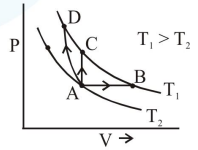# Three different processes that can occur in an ideal monoatomic

Question:

Three different processes that can occur in an ideal monoatomic gas are shown in the $\mathrm{P}$ vs $\mathrm{V}$ diagram. The paths are labelled as $\mathrm{A} \rightarrow \mathrm{B}, \mathrm{A} \rightarrow \mathrm{C}$ and $\mathrm{A} \rightarrow \mathrm{D}$. The change in internal energies during these process are taken as $\mathrm{E}_{\mathrm{AB}}, \mathrm{E}_{\mathrm{AC}}$ and $\mathrm{E}_{\mathrm{AD}}$ and the workdone as $\mathrm{W}_{\mathrm{AB}}$, $\mathrm{W}_{\mathrm{AC}}$ and $\mathrm{W}_{\mathrm{AD}}$. The correct relation between these parameters are :1. $\mathrm{E}_{\mathrm{AB}}=\mathrm{E}_{\mathrm{AC}}=\mathrm{E}_{\mathrm{AD}}, \mathrm{W}_{\mathrm{AB}}>0, \mathrm{~W}_{\mathrm{AC}}=0$$\mathrm{W}_{\mathrm{AD}}>0$

2. $\mathrm{E}_{\mathrm{AB}}<\mathrm{E}_{\mathrm{AC}}<\mathrm{E}_{\mathrm{AD}}, \mathrm{W}_{\mathrm{AB}}>0, \mathrm{~W}_{\mathrm{AC}}>\mathrm{W}_{\mathrm{AD}}$

3. $\mathrm{E}_{\mathrm{AB}}=\mathrm{E}_{\mathrm{AC}}<\mathrm{E}_{\mathrm{AD}}, \mathrm{W}_{\mathrm{AB}}>0, \mathrm{~W}_{\mathrm{AC}}=0$

$\mathrm{~W}_{\mathrm{AD}}<0$

4. $\mathrm{E}_{\mathrm{AB}}>\mathrm{E}_{\mathrm{AC}}>\mathrm{E}_{\mathrm{AD}}, \mathrm{W}_{\mathrm{AB}}<\mathrm{W}_{\mathrm{AC}}<\mathrm{W}_{\mathrm{AD}}$

Correct Option: 1

Solution:

$\Delta \mathrm{U}=\mathrm{nC}_{\mathrm{y}} \Delta \mathrm{T}=$ same

$\mathrm{AB} \rightarrow$ volume is increasing $\Rightarrow \mathrm{W}>0$

$\mathrm{AD} \rightarrow$ volume is decreasing $\Rightarrow \mathrm{W}<0$

$\mathrm{AC} \rightarrow$ volume is constant $\Rightarrow \mathrm{W}=0$